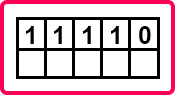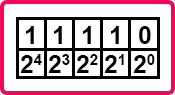# Binary, Octal, Hexadecimal to Decimal Conversion

## Binary, Octal, and Hexadecimal to Decimal Conversion:

In this article, I am going to discuss Binary, Octal, and Hexadecimal to Decimal Conversion with Examples. Please read our previous article where we discussed Decimal to Binary, Octal, and Hexadecimal Conversion with Examples. Now, we will learn how to convert a binary number to a decimal, hexadecimal and octal number. The procedure is the same for all. Let us start with binary to decimal conversion.

##### Binary Number to Decimal Number Conversion:

(11110)2 = (?)10

Here we have a binary number and we want to convert it into a decimal number system. For conversion, we should make a table. This will be easy for conversion. As there are 5 digits in the above binary number so make 5 columns in the table and fill the digits.In the blank row, we will write the power of 2.Now multiply these digits with their corresponding power. So, the equation will be,

= 1 X 24 + 1 X 23 + 1 X 22 + 1 X 21 + 0 X 20

= 16 + 8 + 4 + 2 + 0

= 30

So, 11110 is equivalent to 30 in the decimal system.

(11110)2 = (30)10

Let us take another number that is 101011. Let us convert this binary number into its equivalent decimal number. The procedure is the same. First, we have to create a table then write the digits and in the next row, write the powers of 2.The given number contains 6 digits, so we have filled 6 digits in our table with corresponding powers of 2. So, the equation is,

= 1 X 25 + 0 X 24 + 1 X 23 + 0 X 22 + 1 X 21 + 1 X 20

= 32 + 0 + 8 + 0 + 2 + 1

= 43

Here we get 43 in the decimal number system.

(101011)2 = (43)10

Now before moving further, I will show you an easy and quick method for converting any binary number to a decimal number. This is a very useful method for programmers. If you have any binary number then you should be able to figure out the decimal form of the binary number. So, you should be able to quickly do that. Now let us see how to do that.

Suppose we have a binary number 1010. This is nothing but 10 in the decimal number system. For converting it into decimal, just write the powers of 2 below the digits,We can write the same thing as,Here we have just solved the powers. Now, wherever you are getting one just take that corresponding number. Here 1 Is present on 2 places so the corresponding number are 8 and 2. Now add these numbers,

= 8 + 2

= 10

10 is in the decimal number system. So, this is a simple trick. You can remember the numbers 1,2,4,8,16 and so on. Let us take another number, 101101.Again, add only those numbers which are written corresponding to 1 only. So, the numbers are 32, 8,4, and 1. Let us add these numbers.

= 32 + 8 + 4 + 1

= 45

(101101)2 = (45)10

Let us take another number 1000111.= 64 + 4 + 2 + 1

= 71

(1000111)2 = (71)10

In this way, we can quickly convert binary numbers to the decimal number system. This method will be helpful in your academics as well as in logic designing. Now let us see the conversion of octal numbers to the decimal number system.

##### Octal Number to Decimal Number Conversion:

For octal numbers also, we have to construct a table and write down the octal digits in the table.In the empty row, write the power of 8 from 0.Now multiply these digits with the corresponding power of 8. The equation is,

= 3 X 81 + 6 X 80

= 24 + 6

= 30

(36)8 = (30)10

Let us take another number 46. First, we have to write the digits and then multiply them with the corresponding power of 8.The equation is,

= 4 X 81 + 6 X 80

= 32 + 6

= 38

So, this is the method of converting an octal number to a decimal number. Now let us see the conversion from hexadecimal to the decimal number system.

##### Hexadecimal Number to Decimal Number Conversion:

Let us take a hexadecimal number (1E) 16. We know that the procedure is the same but here the corresponding power will be 16. So let us convert (1E) into a decimal number system. We have to follow the same steps that construct a table then write the digits and then we have to add the multiplication of that digits with their corresponding power.Here we have written the digits as well as the power of 16. Now the equation is,

= 1 X 161 + E X 160

= 1 X 161 + 14 X 1 (E = 14 in decimal number)

= 16 + 14

= 30

(1E) 16 = (30) 10

Let us take another number (2B) 16.Now the equation is,

= 2 X 161 + B X 160

= 2 X 161 + 11 X 1 (B = 11 in decimal number)

= 32 + 11

= 43

(2b)8 = (43)10

So that’s all about the conversion of any number system to a decimal number system. In the upcoming articles, we will see the conversion of octal to hexadecimal and hexadecimal to the octal number system. There you will understand the purpose of the octal and hexadecimal number system.